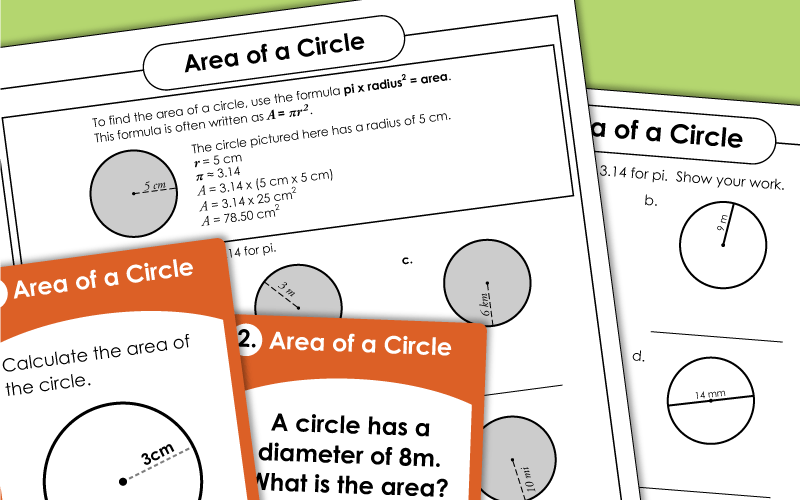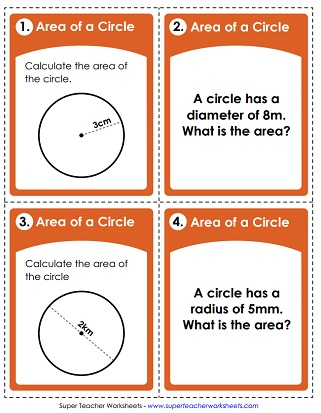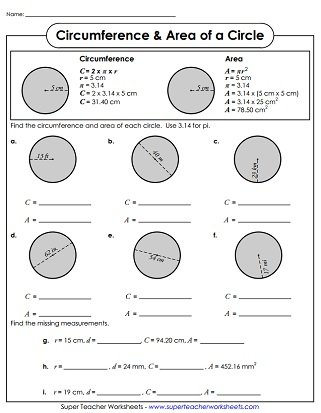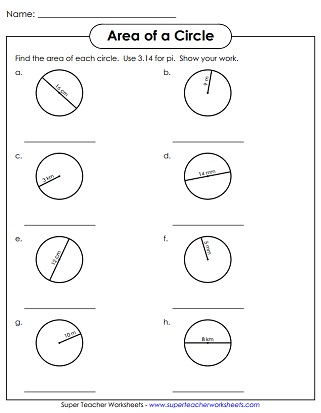# Areas of Circles

This page has a collection of printable (PDF) geometry worksheets for calculating the areas of circles.## Printable Worksheets

Students use the formula to calculate the area of each circle.
This area-of-a-circle worksheet has an explanation and example at the top of the page.
Determine the area of each circle shown. This worksheet includes radius amounts with double digit numbers and areas with decimals.
Students will need to find both the area and circumference for each circular shape.

If you're looking for an alternative to worksheets, try these printable task cards. Each one of the thirty cards in this set has a picture with an "area of a circle" math problem.

These worksheets cover circumferences of circles, and well as introductions to radius and diameter.

Area of Triangles

Find the areas of the triangles using the correct formula.

More Area Worksheets

This page will connect you to worksheets on areas of rectangles, parallelograms, trapezoids, and surface area.

Geometry Worksheets

Here's an index page that will link you to all types of different geometry worksheet topics, including perimeter, plotting points, volume, similar/congruent, polygons, solid shapes, and more.

## Sample Worksheet ImagesMy Account
Site Information